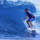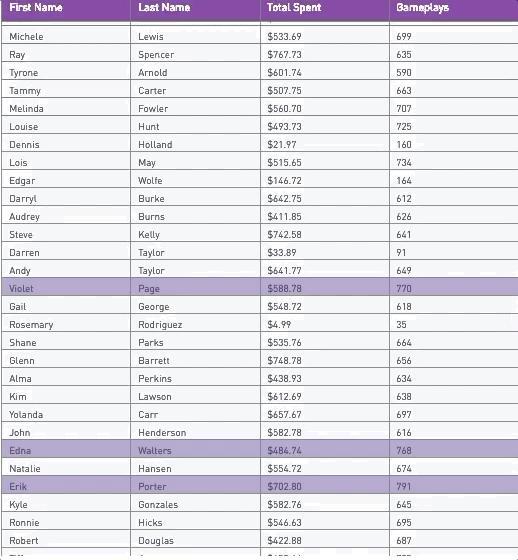# Conditional Formatting On Entire Rows With Plotly

A common request is to apply conditional formatting on entire rows based on a value in a given column. Currently, with Periscope charts, you can only conditionally format columns. Python charts give us much more customizability around conditional formatting, but you sacrifice a lot of the interactivity of Periscope charts. This becomes a problem with viewing larger datasets since a matplotlib table displays as an image and doesn't offer the ability to scroll. Luckily, now with Plotly we can apply more customizable conditional formatting without losing some of the interactivity that you get with Periscope charts.

```import plotly
import plotly.graph_objs as go
import numpy as np

import pandas as pd

first_name = df.first_name.values
last_name = df.last_name.values
total_spent = df.total_spent.values
gameplays = df.gameplays.values

trace = go.Table(columnwidth = [.3,.3,.3,.3],
header=dict(values=['First Name', 'Last Name', 'Total Spent', 'Gameplays'],
fill = dict(color='#8849a5'),
font = dict(color = 'white', size = 12),
align = ['left'] ),
cells=dict(values=[first_name, last_name, total_spent, gameplays],
fill = dict(color=[#unique color for the first column
['#b4a8ce' if val >=750 else '#f5f6f7' for val in gameplays] ]),
align = ['left'] * 5))

data = [trace]

periscope.plotly(data)```